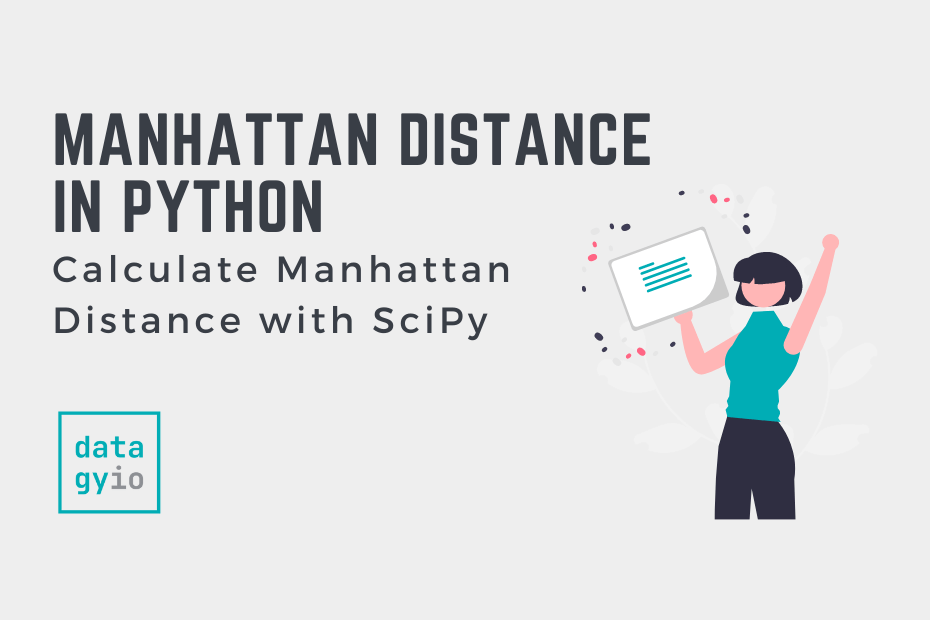# Calculate Manhattan Distance in Python (City Block Distance)In this tutorial, you’ll learn how to use Python to calculate the Manhattan distance. The Manhattan distance is often referred to as the city block distance or the taxi cab distance. The Manhattan distance can be a helpful measure when working with high dimensional datasets.

By the end of this tutorial, you’ll have learned:

• What the Manhattan distance represents
• When the Manhattan distance is used in machine learning
• How to Calculate the Manhattan Distance from Scratch in Python
• How to Use Python SciPy to Calculate the Manhattan Distance

## What is the Manhattan Distance

The Manhattan distance represents the sum of the absolute differences between coordinates of two points. While the Euclidian distance represents the shortest distance, the Manhattan distance represents the distance a taxi cab would have to take (meaning that only right angles can be used).

In a two-dimensional space, the Manhattan distance between two points (x1, y1) and (x2, y2) would be calculated as: `distance = |x2 - x1| + |y2 - y1|`.

In a multi-dimensional space, this formula can be generalized to the formula below:

By its nature, the Manhattan distance will always be equal to or larger than the straight-line distance.

## How is the Manhattan Distance Used in Machine Learning

The Manhattan distance is used frequently in machine learning. Knowing what different distance metrics represent and when each metric may be more appropriate is an important skill.

The Manhattan distance comes with two main advantages:

1. It has been demonstrated to work better with high-dimensional data, especially when compared to the Euclidian distance
2. It is less influenced by outliers than the Euclidian distance. Remember, the Euclidian distance squares the shortest path, meaning that any distances exaggerated by outliers will be exaggerated further.

Keep in mind that machine learning is part science, part art. Because of this, it’s important to understand when one metric may perform better than another, but it’s important to verify this to the uniqueness of your data.

## Calculate the Manhattan Distance from Scratch in Python

Let’s dive into learning how to create a custom function to calculate the Manhattan distance using Python. This is actually a fairly straightforward function to develop, that we can do with pure Python.

Let’s break down what we need to do:

1. Our function needs to take two points in any dimensional-space
2. The function needs to iterate over each point’s dimensions element-wise. The `zip` function is a perfect candidate for this.
3. We need to calculate the absolute difference between the two points
4. We need to add up all the differences

Let’s get started:

``````# Calculating Manhattan Distance from Scratch
def manhattan_distance(point1, point2):
distance = 0
for x1, x2 in zip(point1, point2):
difference = x2 - x1
absolute_difference = abs(difference)
distance += absolute_difference

return distance``````

Let’s break down what we did in the code above:

1. We created a new function that takes two points
2. We instantiated a new variable, `distance`, that starts at 0
3. We iterate over the zip of the points to take the difference, convert it to the absolute value, and use the augmented assignment operator to add it to our distance value

The function breaks down clearly what we want to accomplish, but it’s also a bit pedantic. We can greatly simplify it to the below:

``````# Calculating Manhattan Distance from Scratch
def manhattan_distance(point1, point2):
return sum(abs(value1 - value2) for value1, value2 in zip(point1, point2))``````

Let’s try out our function now to see how we can use it to calculate a Manhattan distance:

``````x1 = (1,2,3,4,5,6)
x2 = (10,20,30,1,2,3)
print(manhattan_distance(x1, x2))

# Returns: 63``````

In the next section, you’ll learn how to calculate the Manhattan distance in Python using the SciPy library.

## Use SciPy to Calculate the Manhattan Distance in Python

The SciPy library makes it incredibly easy to calculate the Manhattan distance in Python. The `scipy.spatial.distance` module comes with a function, `cityblock`, which allows you to calculate the taxi cab distance with ease!

Let’s see how we can import the function:

``from scipy.spatial.distance import cityblock``

It’s important to note, here, that the function isn’t named Manhattan. This can often catch people off guard.

The function takes two points in any dimensional space and returns the Manhattan distance between them. Let’s take a look at our earlier example:

``````# Using scipy to Calculate the Manhattan Distance
from scipy.spatial.distance import cityblock
x1 = [1,2,3,4,5,6]
x2 = [10,20,30,1,2,3]
print(cityblock(x1, x2))

# Returns: 63``````

## Conclusion

In this tutorial, you learned how to calculate the Manhattan, or city block, distance using Python. You learned what the distance represents and how it is used in machine learning. The taxi cab distance provides benefits when working with outliers or higher dimensional data.

Finally, you learned how to calculate the Manhattan from scratch, using a custom function. Then, you learned how to use the SciPy `cityblock` function to calculate the Manhattan distance.